About   Products   Support   Videos   Purchase   Contact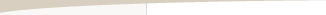Bar Size as an Optimizable Strategy Parameter

by Michael R. Bryant

When developing a trading strategy for intraday data, the starting point is usually the data. Many traders probably have a preferred bar size for day trading, such as 5 minute or 60 minute bars. Sometimes, a trader will report success with an uncommon bar size, such as 3 minute or 135 minute bars. For others, a common approach is to consider a range of bar sizes -- 5, 15, 30, 60 minutes -- and repeat the development process over each bar size.

In this article, I'll adopt a different point of view; namely, that the bar size for an intraday trading strategy should be an optimizable parameter, fundamentally no different than a look-back length for an indicator. I'll show how the optimal bar size for a trading strategy can depend on the characteristics of the strategy, such as how many trades you want to produce and how long the trades last. I'll demonstrate that when the bar size is a dependent property of the strategy rather than an input to the development process, the focus can be on strategy performance rather than on the price data.

Is There an Optimal Bar Size?

For many traders, the bar size may not be a key consideration when building a strategy. Provided there are enough bars within the trading session to support the kind of trades the trader is looking for, it may not seem like the bar size is particularly important. However, the relationship between the type of strategy you're developing and a suitable bar size may not always be obvious. Even in cases where the relationship is fairly clear, the strategy requirements probably only dictate a maximum bar size, not an optimal one. For example, if you want at least five trades per day and expect the trades to run at least 10 bars, that implies at least 50 bars of data per day. For the E-mini S&P, which trades from 8:30 am to 3:15 pm, the session is 405 minutes long. That means the bars can be no larger than 405/50 or 8 minutes. Even in this example, we may not really know how long the trades will tend to last or over how many bars of data the strategy will be flat between trades, so even this maximum value is difficult to estimate.

Another factor is that the bar size will determine the magnitude of the price movements the strategy is aware of and can react to. For example, a 60 minute bar smooths over a lot more price fluctuation than a 5 minute bar. Larger bars are less noisy, but smaller bars permit more trading. It seems logical that the optimal bar size would be the largest size that permits the kind of trading we want. However, since the bar size you choose will in part determine the type of trading results you see, how do you separate the two? As mentioned above, you could repeat everything for a range of bar sizes or, better yet, include the bar size as an optimizable parameter in the strategy development process.

Letting the Bar Size Find its Optimum

To illustrate that the bar size has an optimal value and to show how it can relate to characteristics of the trading strategy, consider the following example. Six months of 1 minute bars of the E-mini S&P 500 futures (ES), ending 6/23/2014, were obtained. Adaptrade Builder was used to build strategies over a population of 100 members with 10 generations. Since the purpose of the study was to examine the relationship between strategy characteristics and the optimal bar size, no trading costs were used, and no out-of-sample testing was performed. Default settings were used except where noted below.

A new feature in Builder (forthcoming in version 1.7.1) was used to include the bar size as a build parameter, as shown below in Fig. 1. When selected, this option treats the bar size for intraday data just like any other strategy parameter. This means the bar size is initialized randomly for each member of the initial population from the range chosen by the user, such as between 5 minutes and 60 minutes. During the build process, the bar size is changed via crossover and mutation as the population is evolved by the genetic programming algorithm. The program uses the 1 minute bars initially provided to construct bars of the required size at each step. After the last generation is completed, the final bar size, which is shown in the build report, is considered the optimal bar size for that strategy.

Two sets of tests were run. In both sets, the number of trades per day was set as a requirement; three different values were tested: 1 - 2 trades per day, 3 - 4 trades per day, and 5 - 7 trades per day. For 1 - 2 trades per day, the number of entries was limited to 5 entries per day. For the other tests, the number of entries was limited to 10 per day. In the first set, a requirement for the average number of bars per trade was set to 10 - 15 bars. The bar size was chosen from the range 5 to 60 minutes in increments of 5 minutes (i.e., 5, 10, 15, 20, etc.). In the second set, the requirement was changed to 20 - 30 bars per trade, and the bar size was chosen from the range 1 to 10 minutes in increments of 1 minute.Figure 1. Trading Logic Options in Builder include the option to try different bar sizes for intraday data.

Fig. 2 shows the full set of build metrics for the first test of the first set. In addition to the requirements ("Build Conditions") for the number of trades per day and number of bars per trade, there are objectives for maximizing net profit and minimizing complexity. The latter was added as a way to add regularization (see, for example, Regularization and Strategy Over-Fitting). Conditions for filtering the results to the "Top Strategies" tables were added so that the strategies that met the build conditions could be isolated.Figure 2. The build metrics for the optimal bar size study include requirements for the number of trades per day and the number of bars per trade.

The testing process consisted of building the population and tabulating the results for each of the six tests (two sets; three tests per set). The bar sizes for the ten most profitable strategies in the Top Strategies tables (i.e., the strategies that met the build conditions) were recorded, and the average bar size and statistical mode (mostly frequently occurring bar size) were calculated.

The results for the first set of tests is shown below in Fig. 3. The results depict the average bar size in minutes for each number of trades per day. The mode value is shown above the bar. The results within the top ten strategies for each test were fairly uniform, with the same optimal bar size occurring in more than half the strategies for all but the first test, where the mode value (25 minutes) occurred four times among the top ten.

The results show that as the number of trades per day increases, the optimal bar size in minutes decreases for a given number of bars per trade. In many of the individual strategies, the optimal bar size was such that the trades utilized most of the chart's bars so that there were very few flat bars. However, not all of the top strategies exhibited this characteristic.Figure 3. Results from the optimal bar size study with 10 - 15 bars per trade show how the optimal bar size (minutes) decreases as the number of trades per day increases.

The results for the second set of tests is shown below in Fig. 4. The results mirror those of the first set, except that with the larger number of bars per trade, the optimal bar size is smaller for each test. Comparing the results between the two figures, it can be seen that the bar size decreased as the number of bars per trade increased for each number of trades per day. Interestingly, the optimal bar size (mode) was the same for the 3 - 4 and 5 - 7 trades per day tests (Fig. 4). While there were certainly strategies where the optimal bar size was less than 3 minutes, only four of the top ten were smaller than this, and all of those were 2 minutes.Figure 4. Results from the optimal bar size study with 20 - 30 bars per trade show how the optimal bar size (minutes) decreases as the number of trades per day increases.

Conclusions

The results from this study show that there was clearly an optimal bar size for each set of test conditions. Generally, the optimal bar size was slightly less than the largest bar size that would fit the average number of trades per day given the average number of bars per trade. This is consistent with the idea presented above that the optimal bar size is as large as possible for the required trades, which was based on the idea that using a bar size smaller than necessary introduces unnecessary noise.

The test sets were based on metrics -- bars per trade and trades per day -- that were expected to be related to the bar size. However, there was no guarantee a priori that there would be an optimal bar size for each test case. It's conceivable that there could have been a wide range of optimal bar sizes for each set of test conditions, either because different types of equally ranked strategy logic favored different bar sizes or because the best logic was indifferent to the bar size. The fact that neither possibility manifested supports the idea that there is an optimal bar size for a specific set of strategy requirements.

On the other hand, it's always possible that if sufficiently non-specific requirements are used, the optimal bar size could vary widely among the top members of the population. For example, I repeated the build process one more time but without the build conditions for the number of trades per day and bars per trade. The only build metrics were the objectives to maximize net profit and minimize complexity. Among the top ten members of the population, the optimal bar size varied from 15 to 40 minutes. It's likely, though, that the bar size was optimal for each strategy's logic, which varied among the top population members. In fact, this is supported by the fact that the two extremes in bar size (15 and 40 minutes) occurred a total of seven times out of 10, suggesting that two different types of strategy logic were similarly successful in the population and that each type of strategy had its own optimal bar size.

When the bar size is included as an optimizable parameter, it's not necessary to choose the bar size beforehand and hope that it works for the kind of strategy you're trying to build. Rather, you can focus on the requirements of the strategy, such as the trading frequency, performance requirements, and so on, and let the bar size take care of itself. This will most likely result in a bar size that better suits the trading logic and helps you meet your strategy development goals.

Mike Bryant

____________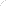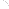Join Our Email List Email: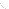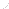For Email Marketing you can trust